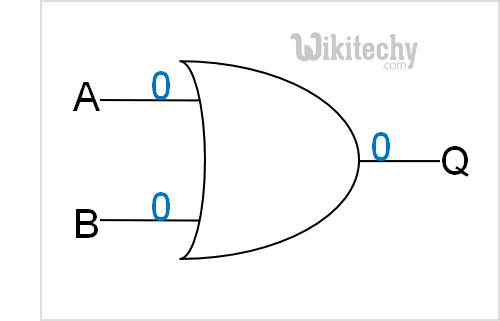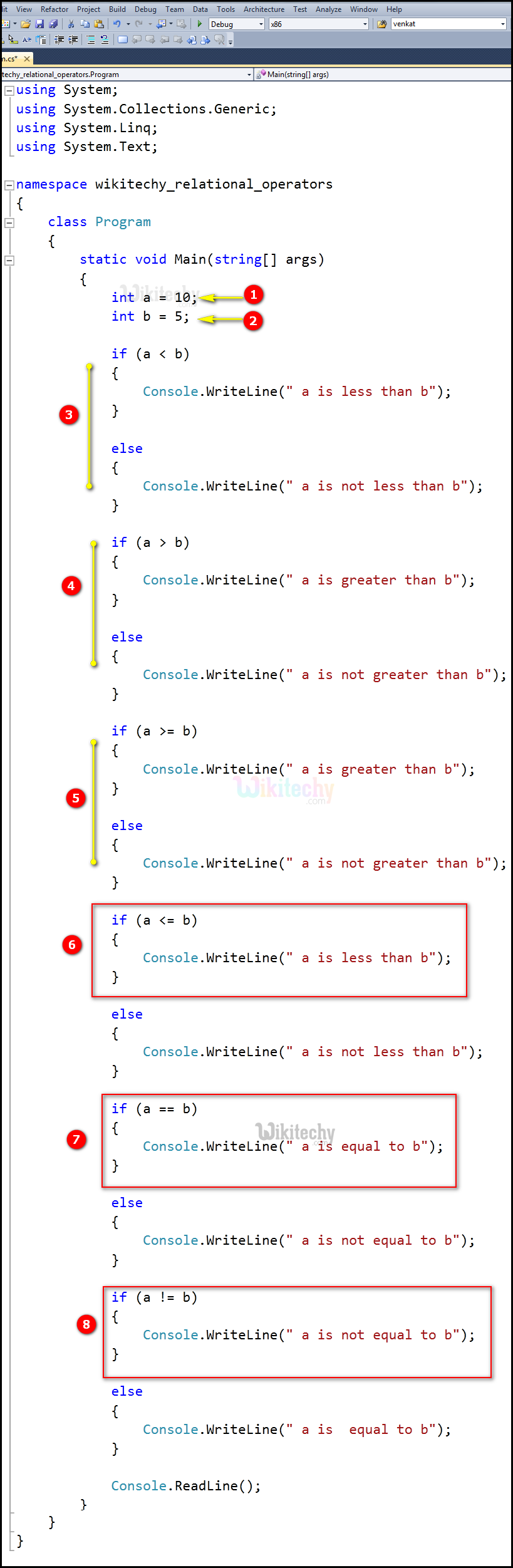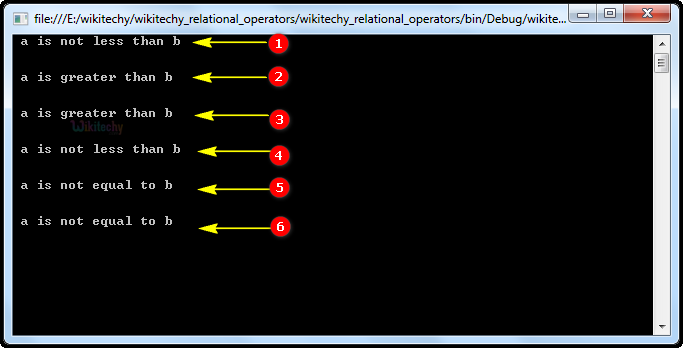# C# Relational Operators - c# - c# tutorial - c# netC# Relational Operators

## What are the Relational Operators in C# ?

• Relational operators are used to find the relation between two variables, i.e. to compare the values of two variables in a C# programming.
• Some of the relational operators are listed below,Relational Operator

S.no Operators Example Description
1 > a > b a is greater than b
2 < a< b a is lesser than b
3 >= a >= b a is greater than or equals to b
4 <= a <= b a is lesser than or equals to b
5 == a == b a is equals to b
6 != a != b a is not equals to b

## Code Explanation:1. Here int a = 10 is an integer variable value as 10.
2. int b = 5 is an integer variable value as 5.
3. In this statement we are using the relational operator lesser than (a < b) for the condition of checking 10 < 5, which will be not satisfied because a=10 and b=5, so the output go to the else statement "false", which will be printed in the statement "a is not less than b".
4. In this statement we are using the relational operator greater than (a > b) for the condition of checking 10 > 5, which will be satisfied because a=10 and b=5, so the output set as "true", which will be printed in the statement "a is greater than b".
5. In this statement we are using the relational operator greater than or equals to (a >= b ) condition of checking here 10>=5 will be satisfied because a=10 and b=5, so the output set as "true", which will be printed in the statement "a is greater than b".
6. In this statement we are using the relational operator lesser than or equal to (a < = b) for the condition of checking 10 <= 5, which will be not satisfied because a=10 and b=5, so the output go to the else statement "false", which will be printed in the statement "a is not less than b".
7. In this statement we are using the relational operator equal to (a == b) for the condition of checking 10 == 5, which will be not satisfied because a=10 and b=5, so the output go to the else statement "false", which will be printed in the statement "a is not equal to b".
8. In this statement we are using the relational operator not equals to ( a != b ) for the condition of checking here 10 != 5 will be satisfied because a=10 and b=5, so the output set as "true" , which will be printed in the statement "a is not equal to b".

## Sample C# examples - Output :1. Here in this output, we have shown the "a is not less than b", which specifies to else statement. Because the less than (a < b) relational operator condition is not satisfied.
2. Here in this output, we have shown the "a is greater than b", which specifies to greater than (a > b) the relational operator condition is satisfied.
3. In this output, we have shown the "a is greater than b", which specifies to greater than or equal to (a >= b) the relational operator condition is satisfied.
4. In this output, we have shown the "a is not less than b", which specifies to else statement. Because the less than (a <= b) relational operator condition is not satisfied.
5. Here in this output, we have shown the "a is not equal to b", which specifies to else statement. Because the equal to (a == b) relational operator condition is satisfied.
6. Here in this output, we have shown the "a is not equal to b", which specifies to not equal to (a != b) relational operator condition is satisfied.